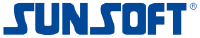# Sun Corp (TSE:6736)

1 570 JPY -47 JPY ( -2.91% )Sun Corp
TSE:6736

## DCF Value

Estimated DCF Value of one6736 stock under the base case scenario is 839.67 JPY. Compared to the current market price of 1 570 JPY, the stock is Overvalued by 47%.

Estimated DCF Value of one TSE:6736 stock is 839.67 JPY. Compared to the current market price of 1 570 JPY, the stock is Overvalued by 47% .

DCF valuation is one of two methods of placing a monetary value on a company; the other is Relative Valuation method. We use a combination of these two methods to calculate the Intrinsic Value of stock as accurately as possible.

Discount Rate
5.73%
Terminal Growth
0%
Growth Period
5 Years
Discount Rate
5.73%
Terminal Growth
0%
Growth Period
5 Years

You can change any inputs, such as future revenue, using Present Value Calculation block.

DCF Valuation FAQ:
6736 DCF Value
Base Case
839.67 JPY
Overvaluation 47%
DCF Value
PriceSun Corp Competitors:
DCF Valuation
6727
Wacom Co Ltd
7999
Mutoh Holdings Co Ltd
AVID
Avid Technology Inc
2369

## DCF Value Calculation

### Capital Structure From Present Value to DCF ValuePresent Value 20.1B JPY Equity Value 20.1B JPY / Shares Outstanding 23.9M 6736 DCF Value 839.67 JPY
Overvalued by 47%

To view the process of calculating the Present Value of Sun Corp' future free cash flow, see the Present Value Calculation block.

## Present Value Calculation

### Discounted Cash Flow Model Present Value of Free Cash FlowRotate your device for better experience.
Currency: JPY

To view the process of deriving the DCF Value of one share from the estimated Present Value, see the DCF Value Calculation block.

## Sensitivity Analysis

### Sensitivity Analysis DCF Value Sensitivity AnalysisAnalyze the possible values of 6736 stock DCF Value given various model inputs such as Revenue Growth, Operating Margin, and Discount Rate.

Similar Stocks

## 6736 Stock DCF Valuation FAQ

What is the DCF value of one 6736 stock?

Estimated DCF Value of one6736 stock under the base case scenario is 839.67 JPY. Compared to the current market price of 1 570 JPY, the stock is Overvalued by 47%.

The true DCF Value lies somewhere between the worst-case and best-case scenario values. This is because the future is not predetermined, and the stock's DCF Value is based almost entirely on the future of the company. Knowing the full range of possible stock intrinsic values gives a complete picture of the investment risks and opportunities.

How was the DCF Value calculated?

1. Present Value Calculation. Using the DCF Operating Model we projectSun Corp's future free cash flow and discount it at a selected discount rate to calculate its Present Value (20.1B JPY).

2. DCF Value Calculation. We use the company's capital structure to calculate the total Equity Value based on the previously computed Present Value of the free cash flow. Dividing the Equity Value by the number of shares outstanding gives us the DCF Value of 839.67 JPY per one6736 share.